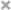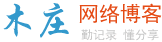# 链表的相关知识整理

## 什么是链表

链表是一种物理存储单元上非连续、非顺序的存储结构，数据元素的逻辑顺序是通过链表中的指针链接次序实现的。链表由一系列结点（链表中每一个元素称为结点）组成，结点可以在运行时动态生成。每个结点包括两个部分：一个是存储数据元素的数据域，另一个是存储下一个结点地址的指针域。

## 链表与数组的区别

回忆下数组的概念 ，所谓数组，是相同数据类型的元素按一定顺序排列的集合。根据概念我们可以知道数组在内存中连续，链表不连续；由于不同的存储方式导致数组静态分配内存，链表动态分配内存，数组元素在栈区，链表元素在堆区；由于数组在内存中连续，我们可以利用下标定位，时间复杂度为O(1)，链表定位元素时间复杂度O(n)；但是由于数组的连续性数组插入或删除元素的时间复杂度O(n)，链表的时间复杂度O(1)。总结一下，数组和链表的区别如下
1.数组静态分配内存，链表动态分配内存
2.数组在内存中连续，链表不连续
3.数组元素在栈区，链表元素在堆区
4.数组利用下标定位，时间复杂度为O(1)，链表定位元素时间复杂度O(n)；
5.数组插入或删除元素的时间复杂度O(n)，链表的时间复杂度O(1)。

## C#实现链表的基本操作

以单链表为例，根据链表的定义我们先定义链表节点的数据结构

 1234567891011121314151617181920212223242526272829303132 `public` `class` `Node``{``    ``private` `T data;``    ``private` `Node next;` `    ``//有参构造函数``    ``//主要用例实例化需要处理的节点用``    ``public` `Node(T item, Node next)``    ``{``        ``data = item;``        ``this``.next = next;``    ``}` `    ``//无参构造函数，用例实例化Node节点``    ``public` `Node()``    ``{``        ``data = ``default``(T);``        ``next = ``null``;``    ``}` `    ``public` `Node Next``    ``{``        ``get` `{ ``return` `next; }``        ``set` `{ ``this``.next = value; }``    ``}` `    ``public` `T Data``    ``{``        ``get` `{ ``return` `data; }``        ``set` `{ ``this``.data = value; }``    ``}``}`

接下来我们来实现链表的操作，构造一个链表，在构造链表里我们定一个头结点的对象，头结点是个很有用的节点，在后续代码中就可以慢慢体会到

 12345678910 `public` `class` `MyLinkList``{``   ``public` `Node Head { ``get``; ``set``; }` `    ``//构造器  ``    ``public` `MyLinkList()``    ``{``        ``Head = ``null``;``    ``}``}`

1.求链表的长度，思路：从头结点向后访问，直到最后一个节点，代码如下

 1234567891011 `public` `int` `Length()`` ``{``     ``var` `p = Head;``     ``int` `len = 0;``     ``while` `(p != ``null``)``     ``{``         ``++len;``         ``p = p.Next;``     ``}``     ``return` `len;`` ``}`

2.清空链表，这个就比较奥简单了，直接将头结点置为null 即可，代码如下

 1234 `public` `void` `Clear()``{``    ``Head = ``null``;``}`

3.同理判断链表是否为空也要用的头结点

 1234567891011 `public` `bool` `IsEmpty()``{``    ``if` `(Head == ``null``)``    ``{``        ``return` `true``;``    ``}``    ``else``    ``{``        ``return` `false``;``    ``}``}`

4.在链表的末尾添加新元素，添加新元素，需要先判断链表是否为空，如果为空我们要给头结点赋值，如果不为空需要修改最后一个节点的next指向，代码如下

 12345678910111213141516 `public` `void` `Append(T item)`` ``{` `     ``if` `(Head == ``null``)``     ``{``         ``Head = ``new` `Node(item, ``null``);``         ``return``;``     ``}``     ``var` `p = ``new` `Node();``     ``p = Head;``     ``while` `(p.Next != ``null``)``     ``{``         ``p = p.Next;``     ``}``     ``p.Next = ``new` `Node(item, ``null``);`` ``}`

5.在单链表的第i个结点的位置前插入一个指定结点，首先需要找到插入的位置，然后修改相邻节点的指向即可， 代码如下

 12345678910111213141516171819202122232425262728293031 `public` `void` `Insert(T item, ``int` `i)``{` `    ``if` `(IsEmpty() || i < 1 || i > GetLength())``    ``{``        ``return``;``    ``}``    ``//如果在第一个位置插入 则只需要将该节点的next 指向head即可``    ``if` `(i == 1)``    ``{``        ``var` `first = ``new` `Node(item, ``null``);``        ``first.Next = Head;``        ``Head = first;``        ``return``;``    ``}` `    ``var` `p = ``new` `Node();``    ``p = Head;``    ``var` `left = ``new` `Node();``    ``var` `right = ``new` `Node();``    ``int` `j = 1;``    ``while` `(p.Next != ``null` `&& j < i)``    ``{``        ``left = p;``        ``right = p.Next;``        ``++j;``    ``}``    ``var` `q = ``new` `Node(item, ``null``);``    ``left.Next = q;``    ``q.Next = right;``}`

6.删除指定节点，先找到要删除的前一个结点，然后修改该结点的next指向即可 代码略。。。。

?　　7.链表还有删除、获取、查找等操作，基本思想都是一样的，就不一一介绍了

## 链表相关的经典题目

1. 求单链表中结点的个数
2. 将单链表反转
3. 查找单链表中的倒数第K个结点（k > 0）
4. 查找单链表的中间结点
5. 从尾到头打印单链表
7. 判断一个单链表中是否有环
8. 判断两个单链表是否相交
9. 求两个单链表相交的第一个节点
10. 已知一个单链表中存在环，求进入环中的第一个节点

c# 一些面试试题的实例教程

asp .net 面试题及答案分享### 评论

• 欢迎访问木庄网络博客
• 可复制：代码框内的文字。
• 方法：Ctrl+C。